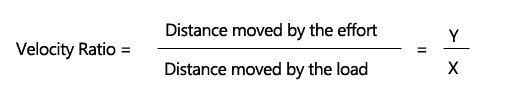# Velocity ratio of machine (Formula)

Define velocity ratio of a machine and how it is determined –
The velocity ratio (V.R.) of a machine is the ratio of distance moved by the effort to distance moved by the load.

Formula –
Velocity Ratio = Distance moved by the effort / Distance moved by the load = y/xThe velocity of given machine can also be determined by assuming the machine to be ideal.